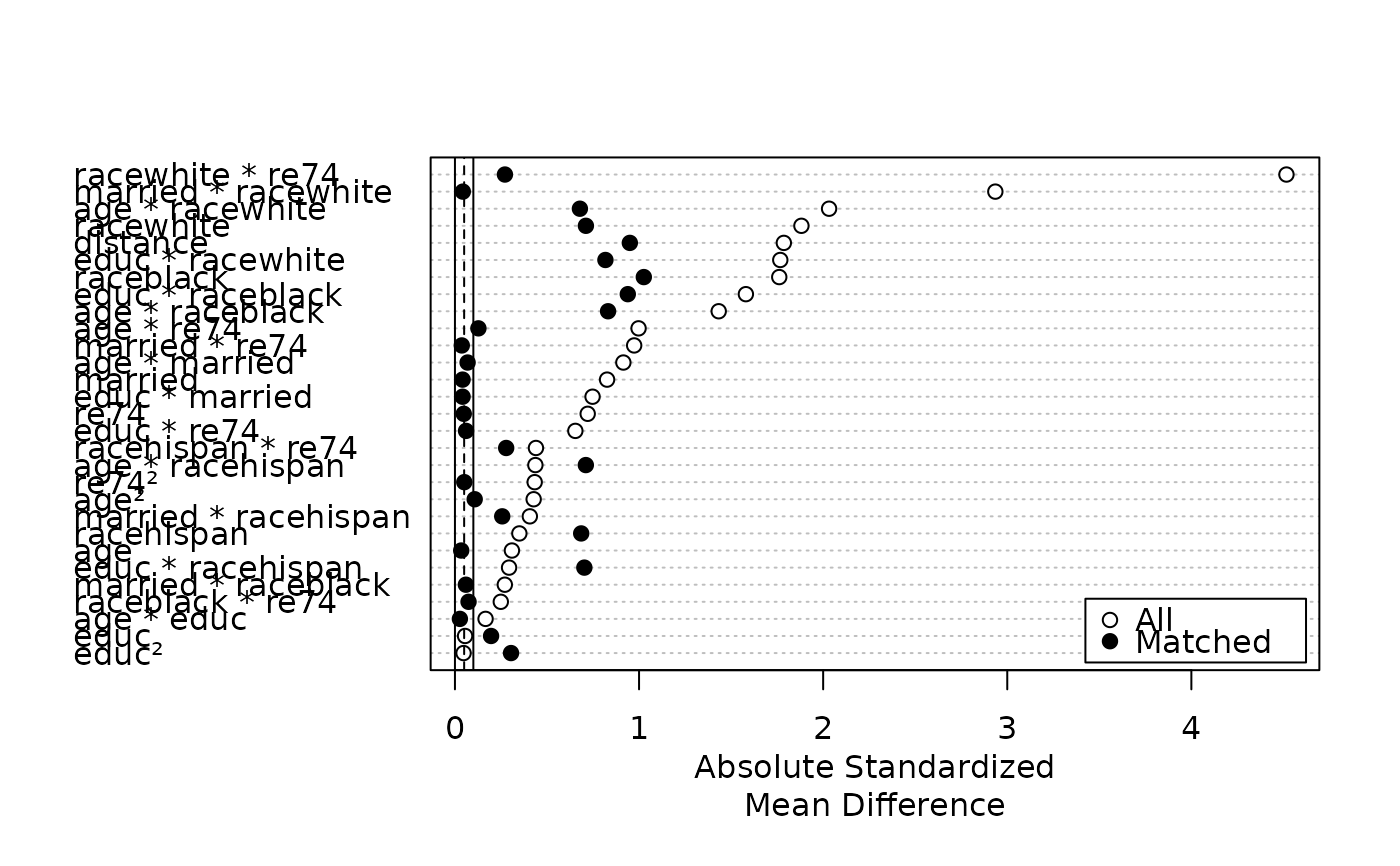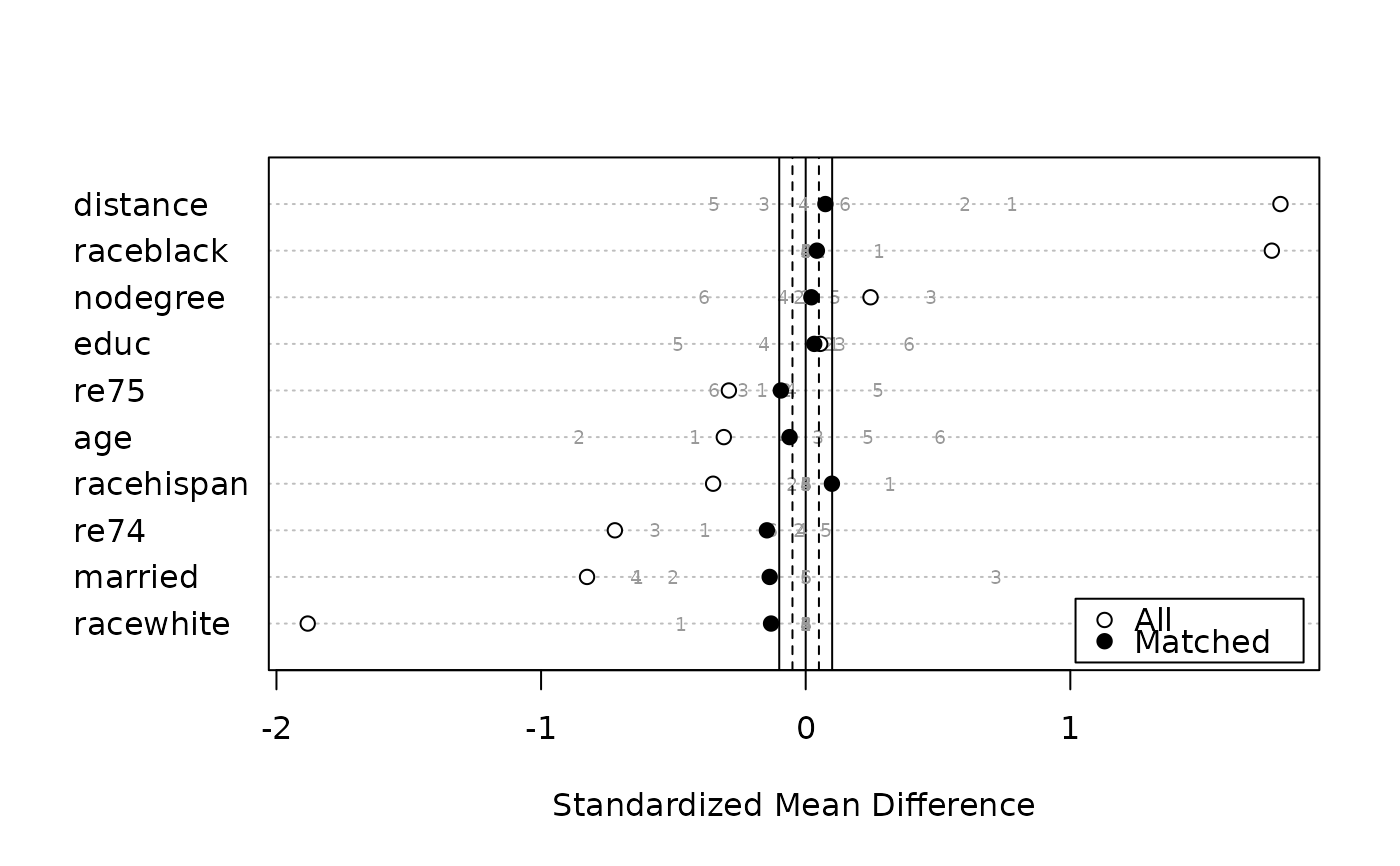Generates a Love plot, which is a dot plot with variable names on the y-axis and standardized mean differences on the x-axis. Each point represents the standardized mean difference of the corresponding covariate in the matched or unmatched sample. Love plots are a simple way to display covariate balance before and after matching. The plots are generated using dotchart() and points().

# S3 method for summary.matchit
plot(
x,
abs = TRUE,
var.order = "data",
threshold = c(0.1, 0.05),
position = "bottomright",
...
)

## Arguments

x

a summary.matchit object; the output of a call to summary.matchit(). The standardize argument must be set to TRUE (which is the default) in the call to summary.

abs

logical; whether the standardized mean differences should be displayed in absolute value (TRUE, default) or not FALSE.

var.order

how the variables should be ordered. Allowable options include "data", ordering the variables as they appear in the summary output; "unmatched", ordered the variables based on their standardized mean differences before matching; "matched", ordered the variables based on their standardized mean differences after matching; and "alphabetical", ordering the variables alphabetically. Default is "data". Abbreviations allowed.

threshold

numeric values at which to place vertical lines indicating a balance threshold. These can make it easier to see for which variables balance has been achieved given a threshold. Multiple values can be supplied to add multiple lines. When abs = FALSE, the lines will be displayed on both sides of zero. The lines are drawn with abline with the linetype (lty) argument corresponding to the order of the entered variables (see options at par()). The default is c(.1, .05) for a solid line (lty = 1) at .1 and a dashed line (lty = 2) at .05, indicating acceptable and good balance, respectively. Enter a value as NA to skip that value of lty (e.g., c(NA, .05) to have only a dashed vertical line at .05).

position

the position of the legend. Should be one of the allowed keyword options supplied to x in legend() (e.g., "right", "bottomright", etc.). Default is "bottomright". Set to NULL for no legend to be included. Note that the legend will cover up points if you are not careful; setting var.order appropriately can help in avoiding this.

...

ignored.

## Value

A plot is displayed, and x is invisibly returned.

## Details

For matching methods other than subclassification, plot.summary.matchit uses x$sum.all[,"Std. Mean Diff."] and x$sum.matched[,"Std. Mean Diff."] as the x-axis values. For subclassification, in addition to points for the unadjusted and aggregate subclass balance, numerals representing balance in individual subclasses are plotted if subclass = TRUE in the call to summary. Aggregate subclass standardized mean differences are taken from x$sum.across[,"Std. Mean Diff."] and the subclass-specific mean differences are taken from x$sum.subclass.

summary.matchit(), dotchart()

cobalt::love.plot() is a more flexible and sophisticated function to make Love plots and is also natively compatible with matchit objects.

Noah Greifer

## Examples


data("lalonde")
m.out <- matchit(treat ~ age + educ + married +
race + re74, data = lalonde,
method = "nearest")
plot(summary(m.out, interactions = TRUE),
var.order = "unmatched")s.out <- matchit(treat ~ age + educ + married +
race + nodegree + re74 + re75,
data = lalonde, method = "subclass")
plot(summary(s.out, subclass = TRUE),
var.order = "unmatched", abs = FALSE)